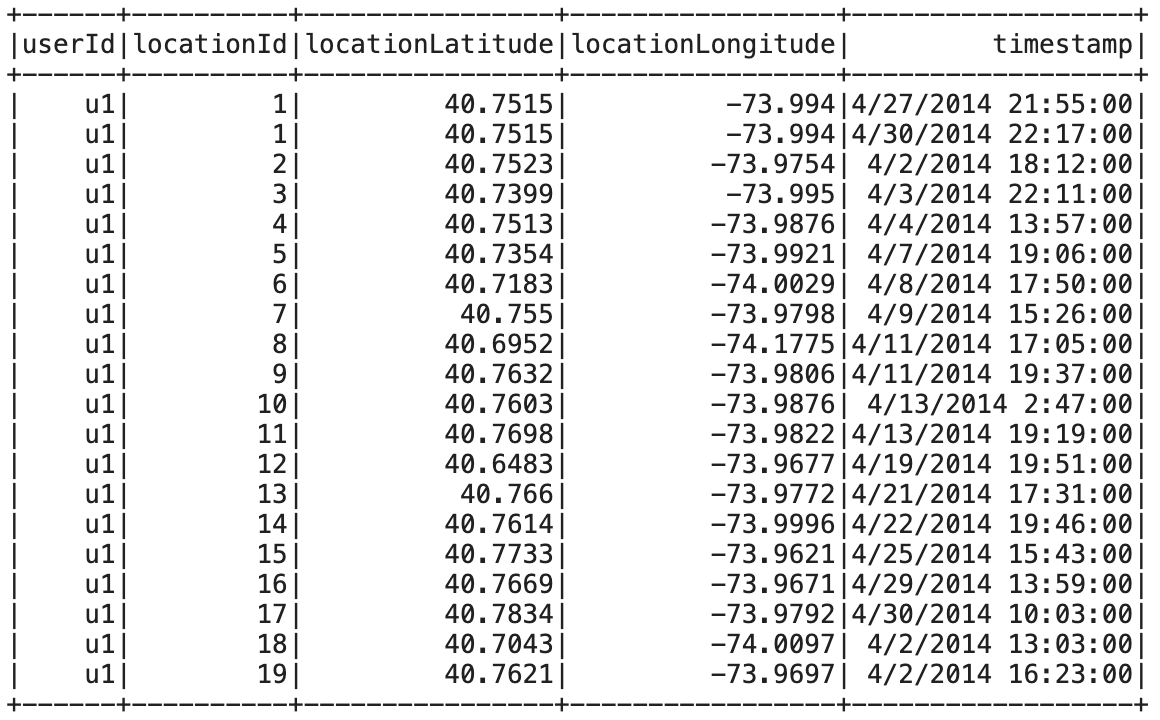# PySpark: compute the radius of gyration

2021, Mar 24

In my line of research, I often have to deal with human behavioral data generated by mobile phones. Mobile phone data has been successfully used to address several research questions in different fields including finance, epidemiology, social network, and human mobility 1.
One source of data that it is possible to obtain from mobile phones is location data, either collected from the antennas through which phone calls are routed, or from GPS sensors. Since we always bring our phones with us, it is possible to get a pretty accurate picture of the mobility behavior of individuals.

### Radius of gyration

A well know metric used to study the characteristic travel distance covered by individuals is the radius of gyration, which measures how far an individual moves around its center of mass 2. It is defined as:

$r_g = \sqrt{\frac{1}{N} \sum_{i \in L} n_i (r_i - r_{cm})^2}$

where:

• $$N$$ is the total number of visits (or total time spent) a particular individual made to all his/her visited locations $$L$$
• $$n_i$$ are the visits (time spent) to location $$i$$
• $$L$$ is the set of visited locations
• $$r_i$$ is the location’s GPS position recorded as latitude and longitude,
• $$r_{cm}$$ is the center of mass of the trajectories defined as:

$r_{cm} = \frac{1}{N} \sum_{i=1}^{N} r_i$

Computing the radius of gyration of thousands of individuals is fairly easy and there are python libraries such as scikit-mobility that do the job for you. Plain python/pandas do not scale very well when you have to compute the radius of gyration for millions or hundreds of millions of individuals. To overcome this limitation, we can use Apache Spark, a distributed data processing framework that can quickly perform tasks on very large data sets in a distributed fashion.

Here the PySpark code to compute the radius of gyration:

w = Window().partitionBy('userId')

radius_df = (gps_data
# number of visits per stop
.groupby('userId', 'locationId')
.agg(F.count(F.lit(1)).alias('n_i'),
F.first('locationLongitude')
.alias('locationLongitude'),
F.first('locationLatitude')
.alias('locationLatitude'))
#compute center of mass (lat/lon) per user
.withColumn('center_lon',
F.avg(F.col('locationLongitude')).over(w))
.withColumn('center_lat',
F.avg(F.col('locationLatitude')).over(w))
# compute total visits
.withColumn('N', F.sum(F.col('n_i')).over(w))
# compute (r_i - r_cm)
.withColumn('distance',
distance(F.col('locationLatitude'),
F.col('locationLongitude'),
F.col('center_lat'),
F.col('center_lon')))
# compute n_i(r_i - r_cm)^2 / N
.withColumn('distance2',
F.col('n_i') * (F.col('distance') * F.col('distance')) / F.col('N'))
# compute sum(n_i(r_i - r_cm)^2)
.groupBy('userId')
.agg(F.sum(F.col('distance2'))
.alias('sum_dist2'))
# square root
.withColumn('radius_gyr', F.sqrt(F.col('sum_dist2')))
.select('userId','radius_gyr')
)


where distance is a function described in a previous post that computes the great-circle distance between two GPS points, and gps_data is a dataframe similar to the one in Fig 1 below.Fig 1. An example of gps data needed to compute the radius of gyration.

You can find the complete code in this [notebook]

1. Blondel, V. D., Decuyper, A., & Krings, G. (2015). “A survey of results on mobile phone datasets analysis.” EPJ data science, 4(1), 10.

2. Gonzalez, M. C., Hidalgo, C. A., & Barabasi, A. L. (2008). “Understanding individual human mobility patterns.” Nature, 453(7196), 779-782.